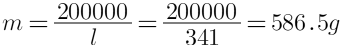### Question 15. Solve the following problems: Liquid ammonia is used in an ice factory for making ice from water. If the water at 20oC is to be converted into 2 kg ice at 0oC, how many grams of ammonia is to be evaporated?

Question 15.

Solve the following problems:
Liquid ammonia is used in an ice factory for making ice from water. If the water at 20oC is to be converted into 2 kg ice at 0oC, how many grams of ammonia is to be evaporated?

(Given: The latent heat of vaporization of ammonia= 341 cal/g)

Given: Latent heat of vapourisation of Ammonia =341 cal/g
Mass of ammonia be= M
Mass of water =2000g
Temperature change, ΔT=20oC-0oC=20oC

latent heat is the heat required by the ammonia for the liquid ammonia to ammonia vapours.

Amount of heat energy released in colling 2 Kg water from 20oC to 0oC
QW=MCΔT

Where
M is the mass of water
C is specific at constant volume
ΔT is the temperature difference

Putting the values in the above formula, we get
Qw=2000×1×20=40000 cal

Amount of heat energy released during the conversion of 2kg of water at 0oC to ice at 0oC
Qi =M×L

Where
M is the mass of the water
L is the latent heat of conversion of water to ice
Putting the values in the above equation, we get
Qi=2000×80=160000 cal

The heat gained by ice due presence of ammonia will be:

Q = m× L=Qi +Qw

Where
m is the mass of the ammonia
L is the latent heat of the ammonia
On solving, we get
m× L=160000+40000=200000 calThus, the process of conversion of 2kg of water to ice will require evaporation of 586.5 g of ammonia